# GMAT Math : DSQ: Calculating the endpoints of a line segment

## Example Questions

### Example Question #1 : Dsq: Calculating The Endpoints Of A Line Segment

Consider segment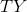with midpoint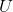at the point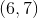.

I) Point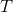has coordinates of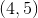.

II) Segmenthas a length ofunits.

What are the coordinates of point?

Statement II is sufficient to answer the question, but statement I is not sufficient to answer the question.

Either statement alone is sufficient to answer the question.

Statement I is sufficient to answer the question, but statement II is not sufficient to answer the question.

Both statements are necessary to answer the question.

Statement I is sufficient to answer the question, but statement II is not sufficient to answer the question.

Explanation:

In this case, we are given the midpoint of a line and asked to find one endpoint.

Statement I gives us the other endpoint. We can use this with midpoint formula (see below) to find our other point.

Midpoint formula:Statment II gives us the length of the line. However, we know nothing about its orientation or slope. Without some clue as to the steepness of the line, we cannot find the coordinates of its endpoints. You might think we can pull of something with distance formula, but there are going to be two unknowns and one equation, so we are out of luck.

So,

Statement I is sufficient to answer the question, but statement II is not sufficient to answer the question.

### Example Question #2 : Dsq: Calculating The Endpoints Of A Line Segment

Find endpoint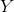given the following:

I) Segment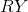has its midpoint at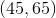.

II) Pointis located on the-axis,points from the origin.

Both statements are needed to answer the question.

Statement I is sufficient to answer the question, but statement II is not sufficient to answer the question.

Statement II is sufficient to answer the question, but statement I is not sufficient to answer the question.

Either statement is sufficient to answer the question.

Both statements are needed to answer the question.

Explanation:

Find endpoint Y given the following:

I) Segment RY has its midpoint at (45,65)

II) Point R is located on the x-axis, 13 points from the origin.

I) Gives us the location of the midpoint of our segment

(45,65)

II) Gives us the location of one endpoint

(13,0)

Use I) and II) to work backwards with midpoint formula to find the other endpoint.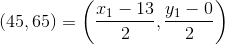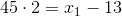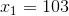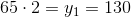So endpointis at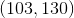.

Therefore, both statements are needed to answer the question.

### Example Question #3 : Dsq: Calculating The Endpoints Of A Line Segment

Consider segment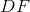I) Endpointis located at the origin

II)has a distance of 36 units

Where is endpointlocated?

Statement II is sufficient to answer the question, but Statement I is not sufficient to answer the question.

Either statement is sufficient to answer the question.

Both statements are needed to answer the question.

Statement I is sufficient to answer the question, but Statement II is not sufficient to answer the question.

Explanation:

To find the endpoint of a segment, we can generally use the midpoint formula; however, in this case we do not have enough information.

I) Gives us one endpoint

II) Gives us the length of DF

The problem is that we don't know the orientation of DF. It could go in infinitely many directions, so we can't find the location ofwithout more information.

### Example Question #4 : Dsq: Calculating The Endpoints Of A Line Segment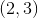is the midpoint of line PQ. What are the coordinates of point P?

(1) Point Q is the origin.

(2) Line PQ is 8 units long.

EACH statement ALONE is sufficient.

Statements (1) and (2) TOGETHER are NOT sufficient.

BOTH statements TOGETHER are sufficient, but NEITHER statement ALONE is sufficient.

Statement (1) ALONE is sufficient, but statement (2) alone is not sufficient.

Statement (2) ALONE is sufficient, but statement (1) alone is not sufficient.

Statement (1) ALONE is sufficient, but statement (2) alone is not sufficient.

Explanation:

The midpoint formula is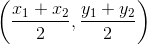,

with statement 1, we know that Q is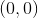and can solve for P: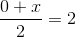and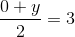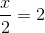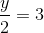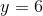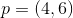Statement 1 alone is sufficient.

Statement 2 doesn't provide enough information to solve for point P.

Tired of practice problems?

Try live online GMAT prep today.